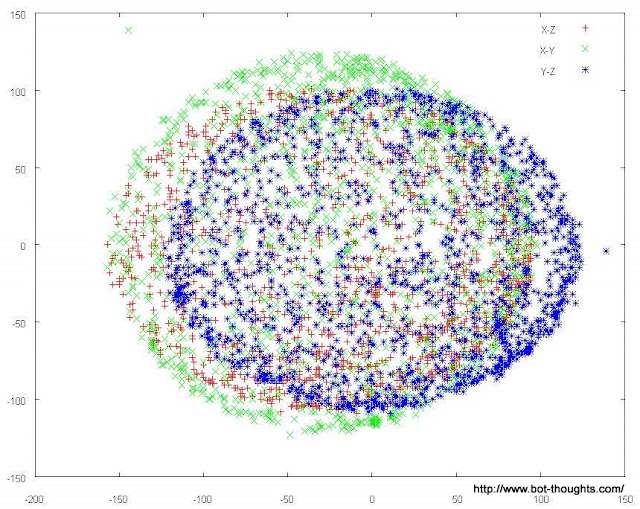Quick and Dirty 3d Compass Calibration

This very simpleton approach improved calibration of my LSM303DLH compass.

Using the LSM303DLH library, first gather uncorrected x, y and z magnetometer data, that is, don't call setOffset() or setScale().

Write a simple program to gather the data and print in CSV format over serial or write to the mbed filesystem.

Throw out any outlier points. You may have to plot the data points in a 3d scatter plot (or three 2d plots) to find the outliers (gnuplot: splot "file.csv" for 3d or plot "file.csv" using 1:2 title "x-y", "" using 1:3 title "x-z", "" using 2:3 title "y-z")Use Excel or a perl (etc) script and find the minimum and maximum values for the x, y and z axes.

Calculate the midpoint between each maximum to get the offset. The offset correction is, of course, the negative of the offset.

Calculate the difference between min and max on each axis and find the highest range of the three. Find the scale for each axis with Max/xmax, Max/ymax, Max/zmax.

Supply these values to the LSM303DLH library via setOffset() and setScale() and now, hopefully, your compass heading accuracy will be improved.

References:

Perl script to compute offset and scale:

#!/usr/bin/perl

open(FIN, "<\$ARGV") || die "can't open: \$ARGV";

\$label{0} = "X";
\$label{1} = "Y";
\$label{2} = "Z";

\$count = 0;
while (<FIN>) {
s/^\s+//;
@data = split(/\s+/);

for (\$i = 0; \$i < 3; \$i++) {
\$sum[\$i] += \$data[\$i];
\$max[\$i] = \$data[\$i] if (\$data[\$i] > \$max[\$i]);
\$min[\$i] = \$data[\$i] if (\$data[\$i] < \$min[\$i]);
}
\$count++;
}
close FIN;

for (\$i = 0; \$i < 3; \$i++) {
\$med[\$i] = (\$max[\$i]+\$min[\$i])/2.0;
printf STDERR "%smax = %6.2f  %smin = %6.2f %soff = %6.2f\n",
\$label{\$i}, \$max[\$i], \$label{\$i}, \$min[\$i], \$label{\$i}, \$med[\$i];
\$max[\$i] -= \$med[\$i];
\$min[\$i] -= \$med[\$i];
\$max = \$max[\$i] if (\$max[\$i] > \$max);
\$min = \$min[\$i] if (\$min[\$i] < \$min);
}

printf STDERR "Max  = %6.2f  Min  = %6.2f\n", \$max, \$min;

for (\$i = 0; \$i < 3; \$i++) {
\$scale[\$i]=\$max/\$max[\$i];
printf STDERR "%ssca = %6.2f  ", \$label{\$i}, \$max/\$max[\$i];
}
printf STDERR "\n";

1 comment on Quick and Dirty 3d Compass Calibration:

Hello Michael,

What do you think of this sensor so far? I am looking for something to use in a water based vehicle and am hesitating between this cheaper solution and a more expensive "all in one" tilt compensated sensor.

Have you done and real world or accuracy testing?

Thanks Serge